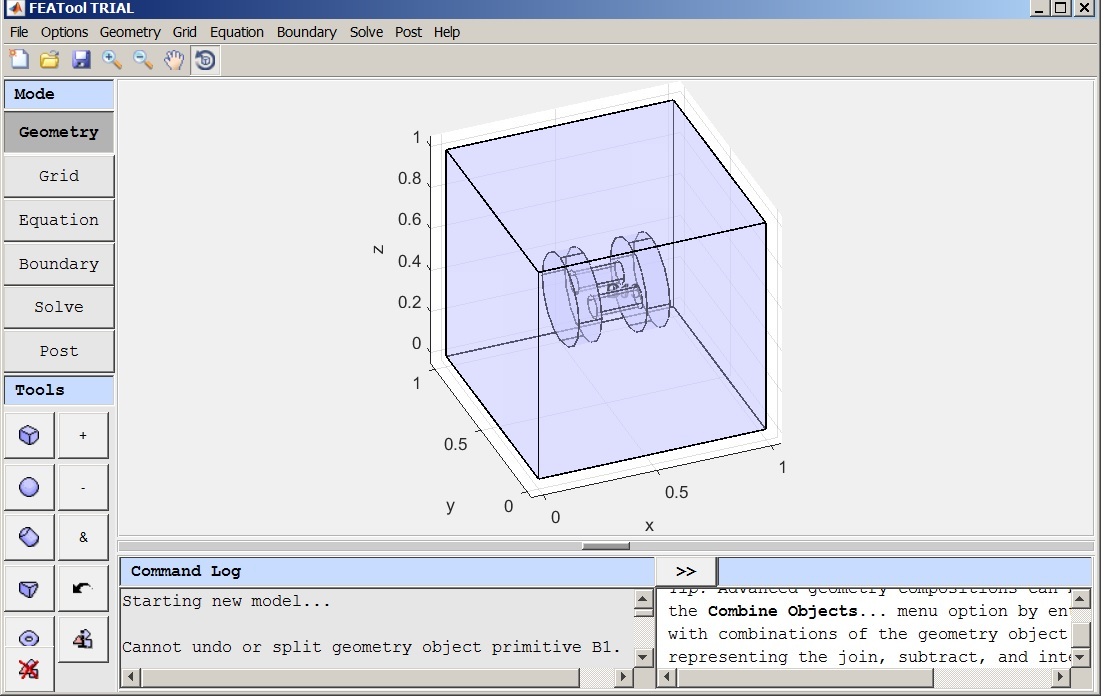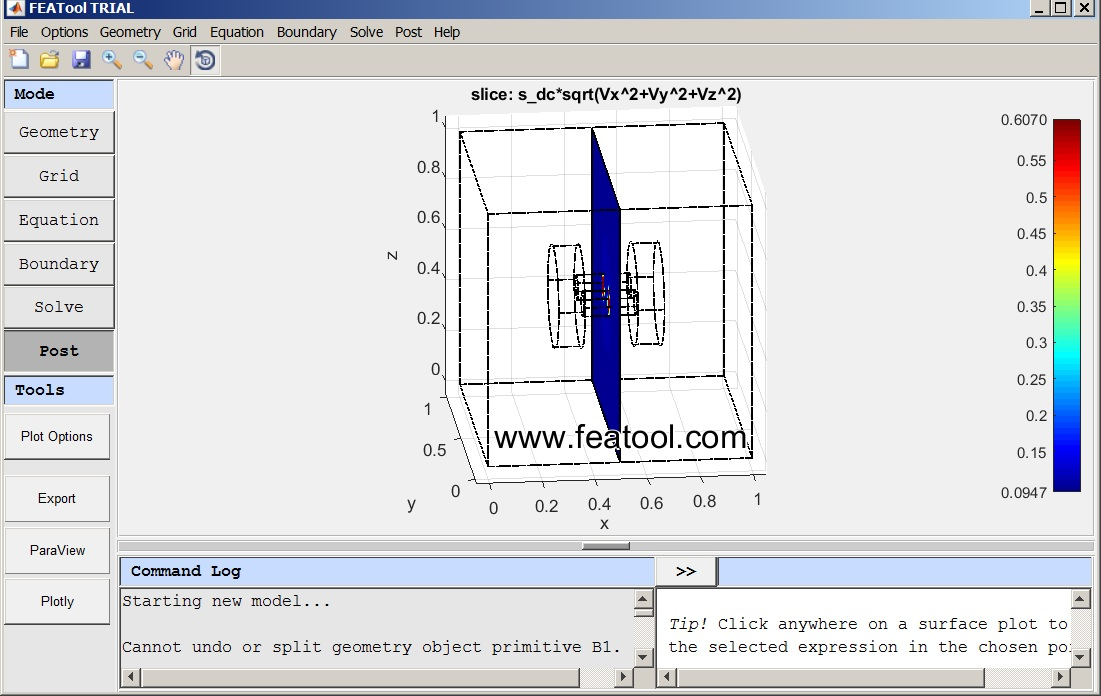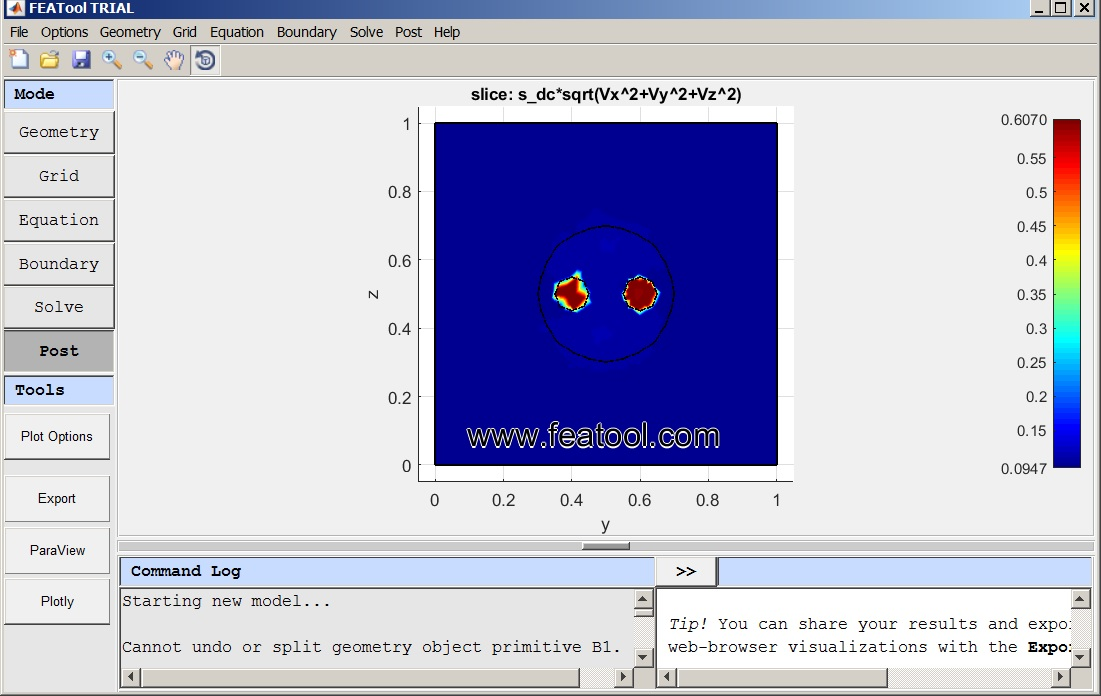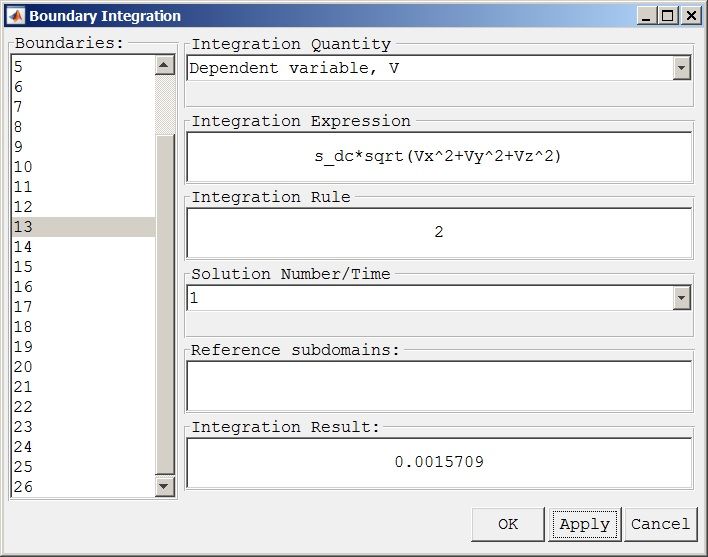# Integration of Current Density over Slice IntersectionClassicListThreaded2 messagesThis post was updated on . The geometry is a 1m cube (subdomain 2) containing a component (subdomain 1) made of 4 joined cylindrical sections. (attached)Boundariess: 5V/0V on the opposite faces of the cube that are perpendicular to the axes of the cylinders. Once solved a YZ plane is cut through the current density (s_dc*sqrt(Vx^2+Vy^2+Vz^2) plot in the center (x=0.5)I want to calculate two currents:    1) the total current flowing into and out-of the cube by integrating the current density over one of the voltage boundaries and    2) the current flowing through each/both of the small connecting cylinders by integrating the current density over the two circular, planar intersections of the cut plane and the two small cylinders.Which tutorials should I consult?  Is there other documentation other than the paragraph from /doc? Boundary/Subdomain Integration Integration of postprocessing expressions over subdomains Subdomain Integration... (calling intsubd) and boundaries Boundary Integration... (calling intbdr) can be performed by selecting the corresponding menu options. The integration dialog box allows for selecting predefined postprocessing or entering user defined expressions in the Integration Expression edit field. Numerical Integration Rule, Solution Number/Time, and Subdomain/Boundary: to integrate over can also be set. The result will be printed in the Integration Result field as well as the command log in the main GUI.Other suggestions? I have been able to perform the integration of the boundaries to get the current in/out of the cube (face 5 and 3). And by selecting the faces of the small cylinders I can get the current into and out-of them at the boundary. (13/14 and 23/24) Here is 13, for example:But I still have not figured out how to do the integration over the slice intersection. Thanks for your help, -Randal cylinders-in-box4.fes